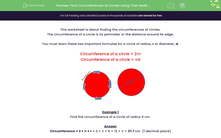# Find the Circumference of a Circle

In this worksheet, students will use formulae to calculate the circumferences of circles given their radii.Key stage:  KS 3

Curriculum topic:   Geometry and Measures

Curriculum subtopic:   Solve Problems Involving Perimeters and Areas of 2D Shapes

Difficulty level:#### Worksheet Overview

This activity is about finding the circumferences of circles.

The circumference of a circle is its perimeter or the distance around its edge.

You need to learn these two important formulae for a circle of radius, r or diameter, d:

Circumference of a circle = 2πr

Circumference of a circle = πd

This is because the diameter of a circle is two times the length of the radius: 2 x r = d

The value of π (pi) is 3.142.... but is an infinite number, so the best way to do any calculations with pi is to use a scientific calculator that has a special π  button.Example 1

Find the circumference of a circle of radius 6 cm.

Circumference = 2 × π × r = 2 × π × 6 = 12 × π = 37.7 cm  (1 decimal place)

Example 2

Find the circumference of a circle of diameter 6 cm.

Circumference = π × d = π × 6 = 18.8 cm  (1 decimal place)

Use a scientific calculator to work out your answer because you can have a more accurate number for pi  (π ).

If you don't have one in the house, you can access one online.

Let's have a go at some questions.

### What is EdPlace?

We're your National Curriculum aligned online education content provider helping each child succeed in English, maths and science from year 1 to GCSE. With an EdPlace account you’ll be able to track and measure progress, helping each child achieve their best. We build confidence and attainment by personalising each child’s learning at a level that suits them.

Get started